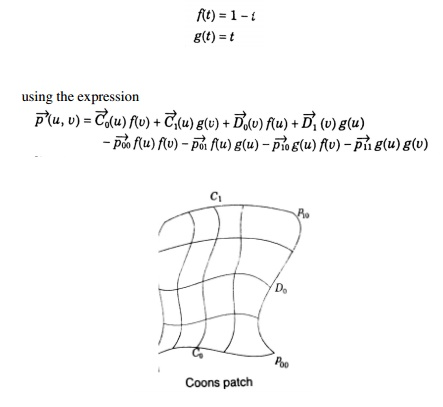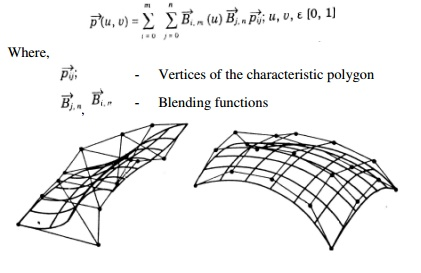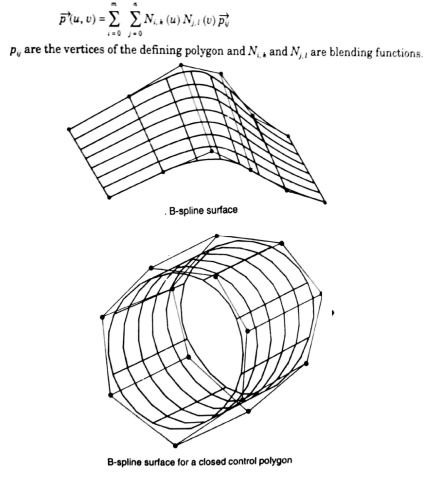# Geometric Modeling

Types Of Curves Used In Geometric Modelling: • Hermite curves • Bezeir curves • B-spline curves • NURBS curves

GEOMETRIC MODELING

PRE-REQUISITE DISCUSSION

CURVE REPRESENTATION

(1) Parametric equation x, y, z coordinates are related by a parametric var iable (u or θ)

(2) Nonparametric equation x, y, z coordinates are related by a function

Example: Circle (2-D)TYPES OF CURVES USED IN GEOMETRIC MODELLING

Hermite curves

Bezeir curves

B-spline curves

NURBS curves

HERMITE CURVESEffect of tangent vector on t he curve’s shapeBEZIER CURVETwo Drawbacks of Bezier CurvesB-SPLINE CURVESNURBS curveAdvantages of B-spline curves and NURBS curve

TECHNIQUES IN SURFACE MODELLING

i.           Surface Patch

ii.           Coons Patch

iii.           Bicubic Patch

iv.          Be’zier Surface

v.           B-Spline Surface

i.           Surface Patch

The patch is the fundamental building block for surfaces. The two variables u and v vary across the patch; the patch may be termed biparametric. The parametric variables often lie in the range 0 to 1. Fixing the value of one of the parametric variables results in a curve on the patch in terms of the other variable (Isoperimetric curve). Figure shows a surface with curves at intervals of u and v of 0 : 1.ii.  Coons Patch

The sculptured surface often involve interpolation across an intersecting mesh of curves that in effect comprise a rectangular grid of patches, each bounded by four boundary curves. The linearly blended coons patch is the simplest for interpolating between such boundary curves. This patch definition technique blends for four boundary curves Ci(u) and Dj(v) and the corner points pij of the patch with the linear blending functions,iii.           Bicubic Patch

The bi-cubic patch is used for surface descriptions defined in terms of point and tangent vector information. The general form of the expressions for a bi-cubic patch is given by:This is a vector equation with 16 unknown parameters kij which can be found by Lagrange interpolation through 4 x 4 grid.

iv.          Be’zier Surface

The Be’zier surface formulation use a characteristic polygon

Points the Bezier surface are given byv.           B-Spline Surfaces

The B-spline surface approximates a characteristics polygon as shown and passes through the corner points of the polygon, where its edges are tangential to the edges of the polygon

This may not happen when the control polygon is closed

A control point of the surface influences the surface only over a limited portion of the parametric space of variables u and v.

The expression for the B-spline surfaces is given byGEOMETRIC MODELLING

Geometric modeling is the starting point of the product design and manufacture process. Functions of Geometric Modeling are:

Design Analysis

Evaluation of area, volume, mass and inertia properties

Interference checking in assemblies

Analysis of tolerance build-up in assemblies

Kinematic analysis of mechanisms and robots

Automatic mesh generation for finite element analysis

Drafting

Automatic planar cross-sectioning

Automatic hidden lines and surface removal

Automatic dimensioning

Automatic creation of exploded views of assemblies

Manufacturing

Parts classification

Process planning

NC data generation and verification

Robot program generation

Production Engineering

Bill of materials

Material requirement

Manufacturing resource requirement

Scheduling

Inspection and quality control

Program generation for inspection machines

Comparison of produced parts with design

PROPERTIES OF A GEOMETRIC MODELING SYSTEM

The geometric model must stay invariant with regard to its location and orientation The solid must have an interior and must not have isolated parts

The solid must be finite and occupy only a finite shape

The application of a transformation or Boolean operation must produce another solid The solid must have a finite number of surfaces which can be described

The boundary of the solid must not be ambiguous

WIRE FRAME MODELING

It uses networks of interconnected lines (wires) to represent the edges of the physical objects being modeled

Also called ‘Edge-vertex’ or ‘stick-figure’ models Two types of wire frame modeling:

1.     2 ½ - D modeling

2.     3 – D modeling

3-D Wire frame models: These are

Simple and easy to create, and they require relatively little computer time and memory; however they do not give a complete description of the part. They contain little information about the surface and volume of the part and cannot distinguish the inside from the outside of part surfaces. They are visually ambiguous as the model can be interpreted in many different ways because in many wire frame models hidden lines cannot be removed. Section property and mass calculations are impossible, since the object has no faces attached to it. It has limited values a basis for manufacture and analysis

2 ½ - D Wire frame models:

Two classes of shape for which a simple wire-frame representation is often adequate are those shapes defined by projecting a plane profile along its normal or by rotating a planar profile about an axis. Such shapes are not two-dimensional, but neither do they require sophisticated three-dimensional schemes for their representation. Such representation is called 2 ½ - D.Study Material, Lecturing Notes, Assignment, Reference, Wiki description explanation, brief detail
Mechanical : Computer Aided Design : Geometric Modeling : Geometric Modeling |